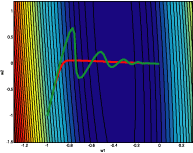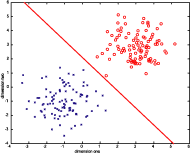# Homework Assignment One

## Problem Two

The following plots are larger versions of the plots in the report. Click on any of them for a larger version. Here is the code that generated these plots.

The following plot shows how the trajectory changes when a pseudo-momentum is added. The red line is the trajectory without momentum, taking 543 iterations, while the green line is the trajectory with momentum, taking 128 iterations. Here is the code that generated these plots.The script used to find the global minimum of the quadratic cost function given in this problem can be found here.

Finally, all of the results for this problem can be generated by calling this single monolithic MATLAB script.

## Problem Five

The following plot shows the discreminating line generated by a classifier using the Least Squares Error method. The code used to generate this plot can be found here.The code used to generate the LMS plots in the report can be found here. These plots are not present here because they are all very similar.

Finally, the code used to generate the Perceptron plots in the report can be found here.